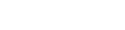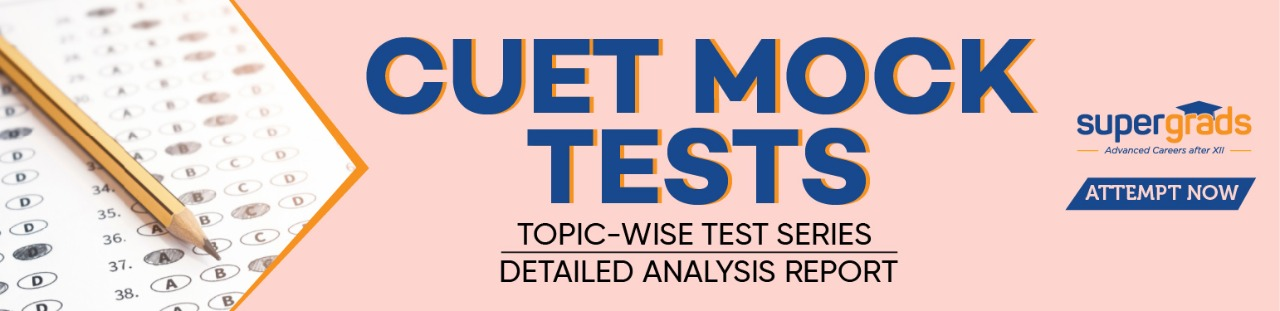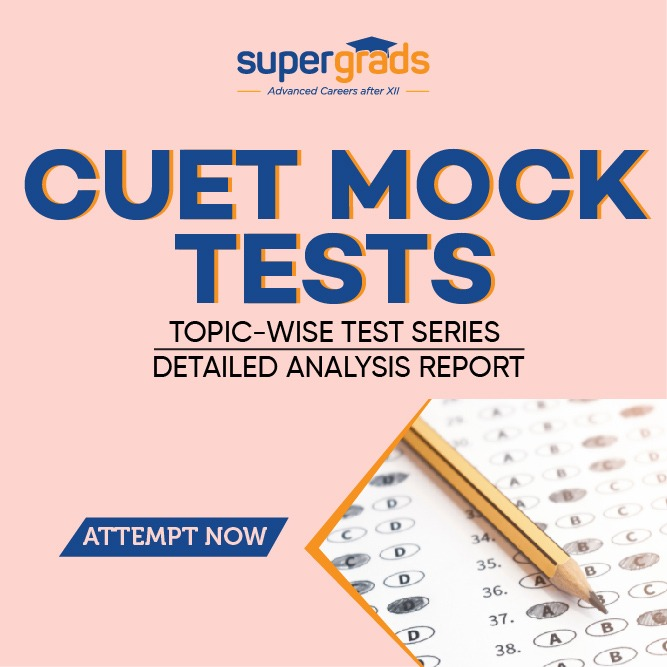• Home
• CUET Phase 2 most im...

# CUET Phase 2 most important topics for maths

Author : Palak Khanna

January 17, 2023

SHARE

Phase 2 of the CUET exam is ongoing, and students looking for important topics for the maths exam are already in a tricky phase.

After the exams, the CUET Answer key for phase 2 and the CUET results will be declared.

So you must appear for the exam with full preparations in order to get the desired college.

## Important Topics for Maths Exam in CUET Phase 2

There are many topics to be covered, and it is always recommended that you cover the maximum of the syllabus. However, if you are unable to do so, you can still have a look at these topics and do well in your exams. Many questions are expected around these topics, so having your concepts cleared will eventually land you at a better rank.

### Pattern for Mathematics and Applied Mathematics

• Section A: 15 Questions are compulsory for both core Math and Applied Math students.
• Section B-1: For Core math students, only 25 out of 35 questions are needed to be done.
• Section B-2: For applied Mathematics students, only 25 out of 35 questions are needed to be done.

### CUET Phase 1 Paper Analysis

 Topics No of Questions Relation and Function 2-4 Question Inverse Trignometry 2 Question Matrices and Determinants 4 Question Continuity, Differentiability, Differentiation 3-5 Question Application of Derivatives 10-12 Question Integration, Definite Integration 4-5 Question Area Under the Curve 2-3 Question Differential Equation 4-5 Question Vector, 3-D 8-10 Question Probability 4-5 Question

Some of the important topics to be covered for Maths Exam in CUET phase 2 are-

Relation and Function- There were 2-4 questions in the phase 1 exam on this topic. So having a look at these sub-topics will be very helpful-

1. Types of relation, reflexive, symmetric, transitive, equivalence, inverse
2. Functions domain and range
3. Types of function- one on one, many one, onto, into, bijection
4. Inverse function

Check out the best online CUET coaching and the mock tests provided.

Inverse Trignometry- Around 2 questions were there on this topic in the Phase 1 exam, so it is expected to repeat in phase 2 as well. Make sure to have a look at these sub-topics-

1. Inverse Function: Domain and Range
2. Find value of x and y.
3. Formula based questions basic.Matrices and Determinants- A total of 4 questions were there in the phase 1 exam, so this is an important topic. Have a look at the important sub-topics-

1. Symmetric and skew symmetric
2. Transpose of matrix
3. Singular Matrix
4. Adjoint of matrix, Inverse of matrix
5. Basic properties based questions on determinants
6. Area of triangle, collinearty
7. Solution of Equation

Read More: Exam pattern for CUET exam

Continuity, Differentiability, Differentiation- This topic can contain 3-5 questions based on the phase 1 exam. So these sub-topics become important to look at-

1. Graph based questions, algebra of continuity
2. Continuity of a point
3. Differentiation of basic, log type question, parametric
4. Differentiation of function w.r.t. other function
5. Differentiation of question w.r.t. point

Application of Derivatives- This topic here is very important as around 10 questions are expected from these sub-topics-

1. Tangent and Normal :Slope of Tangent, Slope of Parallel to Line, Finding of unknown point.
2. Increasing and Decreasing function, Interval
3. Maxima and Minima
4. Approximation do only (2-4 question Less Chance)
5. Rate of Change do only (2-4 question Less Chance)
6. Rolle's Theorem and LMVT Less Chance

Integration, Definite Integration- This is also an important topic expected to have 4-5 questions from these sub-topics-

1. Substitution, Partial Fraction
2. Integration of ex(f(x)+f(x))dx
3. Definite Property a to b or-a to a

LPP (Linear Programming)-

1. Bounded LPP and Unbound LPP
2. Only Z max or Z min
3. Given Graphs find Z max and Z minArea Under the Curve and Differential Equation-

1. Area: Between Curve and Line
2. Area b/w Two curve Parabola
3. Finding Order and Degree
4. Formation of D.E.
5. Homogeneous Equation, Linear Equation, I.Factor
6. Lengthy Question if case study

Read More: Syllabus for CUET Exam

Vector, 3-D- This topic can contain questions up to 10, so preparing for this will include all the sub-topics in this chapter.

1. Angle between 2 vectors
2. Co-planetary conditions
3. Area of the triangle
4. Area of the parellelogram
5. Direction Cosine
6. Perpendicular distance of point from line
7. Questions from plane

Probability- These sub-topics are very important to the topic-

1. Conditional Probability
2. Independent Event
3. Vein Diagram Based
4. Bayes Theorem
5. Probability Distribution Find value of unknown, Mean and Variance Binomial Distribution

There is hardly any chance that you can get the exact same question from the NCERT book.
No, definitely not; you should study and cover all the topics as this is just an analysis of previous papers. Questions may vary in the upcoming exams.
These are the topics that can boost your rank for the best rank, you should cover all the topics.
If you have not selected the subject during the application, you can skip it, but not if you have selected it during the application process.
You will have to attempt 40 out of 50 questions in a domain-based test.
• Home
• CUET Phase 2 most im...

# CUET Phase 2 most important topics for maths

Author : Palak Khanna

Updated On : January 17, 2023

SHARE

Phase 2 of the CUET exam is ongoing, and students looking for important topics for the maths exam are already in a tricky phase.

After the exams, the CUET Answer key for phase 2 and the CUET results will be declared.

So you must appear for the exam with full preparations in order to get the desired college.

## Important Topics for Maths Exam in CUET Phase 2

There are many topics to be covered, and it is always recommended that you cover the maximum of the syllabus. However, if you are unable to do so, you can still have a look at these topics and do well in your exams. Many questions are expected around these topics, so having your concepts cleared will eventually land you at a better rank.

### Pattern for Mathematics and Applied Mathematics

• Section A: 15 Questions are compulsory for both core Math and Applied Math students.
• Section B-1: For Core math students, only 25 out of 35 questions are needed to be done.
• Section B-2: For applied Mathematics students, only 25 out of 35 questions are needed to be done.

### CUET Phase 1 Paper Analysis

 Topics No of Questions Relation and Function 2-4 Question Inverse Trignometry 2 Question Matrices and Determinants 4 Question Continuity, Differentiability, Differentiation 3-5 Question Application of Derivatives 10-12 Question Integration, Definite Integration 4-5 Question Area Under the Curve 2-3 Question Differential Equation 4-5 Question Vector, 3-D 8-10 Question Probability 4-5 Question

Some of the important topics to be covered for Maths Exam in CUET phase 2 are-

Relation and Function- There were 2-4 questions in the phase 1 exam on this topic. So having a look at these sub-topics will be very helpful-

1. Types of relation, reflexive, symmetric, transitive, equivalence, inverse
2. Functions domain and range
3. Types of function- one on one, many one, onto, into, bijection
4. Inverse function

Check out the best online CUET coaching and the mock tests provided.

Inverse Trignometry- Around 2 questions were there on this topic in the Phase 1 exam, so it is expected to repeat in phase 2 as well. Make sure to have a look at these sub-topics-

1. Inverse Function: Domain and Range
2. Find value of x and y.
3. Formula based questions basic.Matrices and Determinants- A total of 4 questions were there in the phase 1 exam, so this is an important topic. Have a look at the important sub-topics-

1. Symmetric and skew symmetric
2. Transpose of matrix
3. Singular Matrix
4. Adjoint of matrix, Inverse of matrix
5. Basic properties based questions on determinants
6. Area of triangle, collinearty
7. Solution of Equation

Read More: Exam pattern for CUET exam

Continuity, Differentiability, Differentiation- This topic can contain 3-5 questions based on the phase 1 exam. So these sub-topics become important to look at-

1. Graph based questions, algebra of continuity
2. Continuity of a point
3. Differentiation of basic, log type question, parametric
4. Differentiation of function w.r.t. other function
5. Differentiation of question w.r.t. point

Application of Derivatives- This topic here is very important as around 10 questions are expected from these sub-topics-

1. Tangent and Normal :Slope of Tangent, Slope of Parallel to Line, Finding of unknown point.
2. Increasing and Decreasing function, Interval
3. Maxima and Minima
4. Approximation do only (2-4 question Less Chance)
5. Rate of Change do only (2-4 question Less Chance)
6. Rolle's Theorem and LMVT Less Chance

Integration, Definite Integration- This is also an important topic expected to have 4-5 questions from these sub-topics-

1. Substitution, Partial Fraction
2. Integration of ex(f(x)+f(x))dx
3. Definite Property a to b or-a to a

LPP (Linear Programming)-

1. Bounded LPP and Unbound LPP
2. Only Z max or Z min
3. Given Graphs find Z max and Z minArea Under the Curve and Differential Equation-

1. Area: Between Curve and Line
2. Area b/w Two curve Parabola
3. Finding Order and Degree
4. Formation of D.E.
5. Homogeneous Equation, Linear Equation, I.Factor
6. Lengthy Question if case study

Read More: Syllabus for CUET Exam

Vector, 3-D- This topic can contain questions up to 10, so preparing for this will include all the sub-topics in this chapter.

1. Angle between 2 vectors
2. Co-planetary conditions
3. Area of the triangle
4. Area of the parellelogram
5. Direction Cosine
6. Perpendicular distance of point from line
7. Questions from plane

Probability- These sub-topics are very important to the topic-

1. Conditional Probability
2. Independent Event
3. Vein Diagram Based
4. Bayes Theorem
5. Probability Distribution Find value of unknown, Mean and Variance Binomial Distribution

There is hardly any chance that you can get the exact same question from the NCERT book.
No, definitely not; you should study and cover all the topics as this is just an analysis of previous papers. Questions may vary in the upcoming exams.
These are the topics that can boost your rank for the best rank, you should cover all the topics.
If you have not selected the subject during the application, you can skip it, but not if you have selected it during the application process.
You will have to attempt 40 out of 50 questions in a domain-based test.